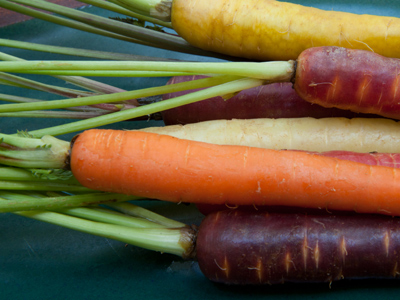Farmer O'Nions sells his carrots in sacks of 120.

# Level 5-6 Numbers - Long Multiplication and Division

Multiplication and division - don't you just love them? Especially long multiplication and long division! Well, whether you like them or not, they play an important part in KS3 Maths so it's best that you understand them.

To do long multiplication we work out the 10s, 100s and 1,000s separately. So, to calculate 154 x 23 first multiply 154 x 3. Then work out 154 x 2 and put a 0 on the end. Finally, line up your two answers and add the top figures to the bottom ones. You should have 462 and 3,080 which total 3,542.

Long division works in a similar way. To calculate 3,542 ÷ 23 first of all work out 35 ÷ 23 = 1 remainder 12. Any remainders become the first digits in the next number to divide. So, next we divide 124 (the 4 is the 3rd digit in 3,542) by 23 and get 5 remainder 9. Finally we divide 92 (the remainder with the 2 from 3,542) by 23 to get 4. So, our answer is 154.

Hopefully all that made sense! Now try some long division and multiplication yourself!

1.
What is 1,000 / 8?
120
125
130
135
10 ÷ 8 = 1r2, 20 ÷ 8 = 2r4 and 40 ÷ 8 = 5 so the answer is 125
2.
Anne has a collection of 483 stamps. Two friends want the same hobby so Anne shares her stamps between the three of them. How many does each person get?
151
155
157
161
483 / 3 = 161
3.
A train carries 107 seated passengers. At Waterloo station there are 33 trains. If the trains are full, how many seated passengers will there be?
3,531
3,786
3,855
4,000
Remember to check your answer by dividing it by 107 (to get 33) or 33 (to get 107)
4.
What is 234 x 87?
20,358
20,538
20,583
20,835
4 x 7 = 28 so you know that the correct answer will end in 8
5.
The latest best-selling novel is 314 pages long. A bookstore orders 840 copies. How many pages will there be?
240,650
256,781
261,009
263,760
That's a lot of pages!
6.
Farmer O'Nions sells his carrots in sacks of 120. A restaurant is doing a carrot soup special and requires 1,000 carrots. How many sacks will they order?
8
9
10
11
1,000 / 120 is more than 8, therefore 9 sacks are needed
7.
What is the remainder when 550 is divided by 9?
1
2
5
7
550 divided by 9 equals 61.1111... Ignore the decimal part and calculate 9 x 61 = 549. Thus, 550 - 549 =1. This method will always give you the whole number remainder in problems like this
8.
Pens are sold in boxes of 85. A shop orders 63 boxes. How many pens does the shop receive?
4,800
4,863
5,355
5,638
63 boxes of 85 pens. The calculation is 63 x 85
9.
Mr Picasso buys 287 paintbrushes for his 41 art students. How many paintbrushes does each student get?
4
7
9
11
7 x 41 = 287
10.
What is 1,445 / 17?
80
85
90
95
You can also multiply your answer by 17 to check it's correct. 85 x 17 = 1,445